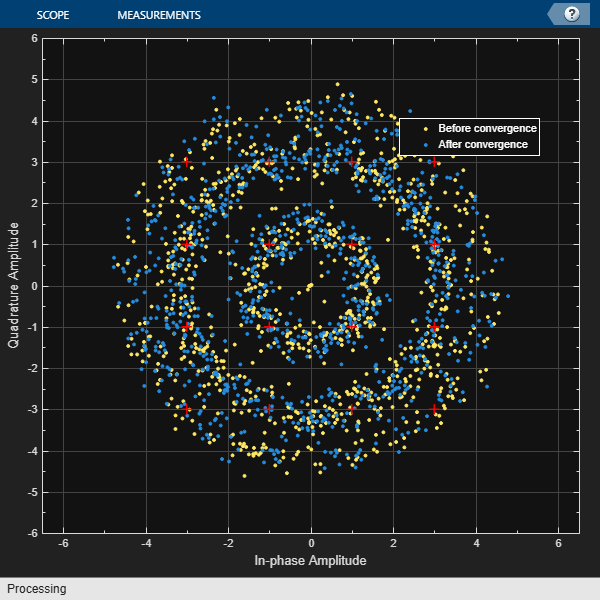# Correct Phase and Frequency Offset for 16-QAM Using Coarse and Fine Synchronization

Compensation of significant phase and frequency offsets for a 16-QAM signal in an AWGN channel is accomplished in two steps. First, correct the coarse frequency offset using the estimate provided by the coarse frequency compensator, and then fine-tune the correction using carrier synchronization. Because of the coarse frequency correction, the carrier synchronizer converges quickly even though the normalized bandwidth is set to a low value. Lower normalized bandwidth values enable better correction for small residual carrier offsets. After applying phase and frequency offset corrections to the received signal, resolve phase ambiguity using the preambles.

Define the simulation parameters.

```fs = 10000; % Sample rate (Hz) sps = 4; % Samples per symbol M = 16; % Modulation order k = log2(M); % Bits per symbol rng(1996) % Set seed for repeatable results barker = comm.BarkerCode(... 'Length',13,'SamplesPerFrame',13); % For preamble msgLen = 1e4; numFrames = 10; frameLen = msgLen/numFrames; ```

Generate data payloads and add the preamble to each frame. The preamble is later used for phase ambiguity resolution.

```preamble = (1+barker())/2; % Length 13, unipolar data = zeros(msgLen, 1); for idx = 1 : numFrames payload = randi([0 M-1],frameLen-barker.Length,1); data((idx-1)*frameLen + (1:frameLen)) = [preamble; payload]; end```

Create a System object for the transmit pulse shape filtering, the receive pulse shape filtering, the QAM coarse frequency compensation, the carrier synchronization, and a constellation diagram.

```txFilter = comm.RaisedCosineTransmitFilter( ... 'OutputSamplesPerSymbol',sps); rxFilter = comm.RaisedCosineReceiveFilter(... 'InputSamplesPerSymbol',sps,'DecimationFactor',sps); coarse = comm.CoarseFrequencyCompensator('SampleRate',fs, ... 'FrequencyResolution',10); fine = comm.CarrierSynchronizer( ... 'DampingFactor',0.4,'NormalizedLoopBandwidth',0.001, ... 'SamplesPerSymbol',1,'Modulation','QAM'); axislimits = [-6 6]; constDiagram = comm.ConstellationDiagram('ReferenceConstellation',qammod(0:M-1,M), ... 'ChannelNames',{'Before convergence','After convergence'}, ... 'ShowLegend',true,'XLimits',axislimits,'YLimits',axislimits);```

Also create a System object for the AWGN channel, and the phase and frequency offset to add impairments to the signal. A phase offset greater than 90 degrees is added to induce a phase ambiguity that results in a constellation quadrant shift.

```ebn0 = 8; freqoffset = 110; phaseoffset = 110; awgnChannel = comm.AWGNChannel('EbNo',ebn0, ... 'BitsPerSymbol',k,'SamplesPerSymbol',sps); pfo = comm.PhaseFrequencyOffset('FrequencyOffset',freqoffset, ... 'PhaseOffset',phaseoffset,'SampleRate',fs);```

Generate random data symbols, apply 16-QAM modulation, and pass the modulated signal through the transmit pulse shaping filter.

```txMod = qammod(data,M); txSig = txFilter(txMod);```

Apply phase and frequency offsets using the `pfo` System object, and then pass the signal through an AWGN channel to add white Gaussian noise.

```txSigOffset = pfo(txSig); rxSig = awgnChannel(txSigOffset);```

The coarse frequency compensator System object provides a rough correction for the frequency offset. For the conditions in this example, correcting the frequency offset of the received signal correction to within 10 Hz of the transmitted signal is sufficient.

`syncCoarse = coarse(rxSig);`

Pass the signal through the receive pulse shaping filter, and apply fine frequency correction.

`rxFiltSig = fine(rxFilter(syncCoarse));`

Display the constellation diagram of the first and last 1000 symbols in the signal. Before convergence of the synchronization loop, the spiral nature of the diagram indicates that the frequency offset is not corrected. After the carrier synchronizer has converged to a solution, the symbols are aligned with the reference constellation.

`constDiagram([rxFiltSig(1:1000) rxFiltSig(9001:end)])`Demodulate the signal. Account for the signal delay caused by the transmit and receive filters to align the received data with the transmitted data. Compute and display the total bit errors and BER. When checking the bit errors, use the later portion of the received signal to be sure the synchronization loop has converged.

```rxData = qamdemod(rxFiltSig,M); delay = (txFilter.FilterSpanInSymbols + rxFilter.FilterSpanInSymbols) / 2; idxSync = 2000; % Check BER for the received signal after the synchronization loop has converged [syncDataTtlErr,syncDataBER] = biterr(data(idxSync:end-delay),rxData(idxSync+delay:end))```
```syncDataTtlErr = 16116 ```
```syncDataBER = 0.5042 ```

Depending on the random data used, there may be bit errors resulting from phase ambiguity in the received signal after the synchronization loop converges and locks. In this case, you can use the preamble to determine and then remove the phase ambiguity from the synchronized signal to reduce bit errors. If phase ambiguity is minimal, the number of bit errors may be unchanged.

```idx = 9000 + (1:barker.Length); phOffset = angle(txMod(idx) .* conj(rxFiltSig(idx+delay))); phOffsetEst = mean(phOffset); disp(['Phase offset = ',num2str(rad2deg(phOffsetEst)),' degrees'])```
```Phase offset = -90.1401 degrees ```
`resPhzSig = exp(1i*phOffsetEst) * rxFiltSig;`

Demodulate the signal after resolving the phase ambiguity. Recompute the total bit errors and BER.

```resPhzData = qamdemod(resPhzSig,M); [resPhzTtlErr,resPhzBER] = biterr(data(idxSync:end-delay),resPhzData(idxSync+delay:end))```
```resPhzTtlErr = 5 ```
```resPhzBER = 1.5643e-04 ```